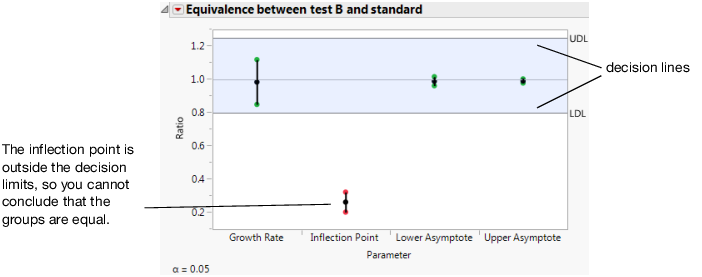Publication date: 11/10/2021

## Equivalence Test

The Equivalence Test report gives an analysis for testing the equivalence of models across levels of the grouping variable (Figure 14.12). After selecting the option, you specify the level of the grouping variable that you want to test against every other level. There is a report for every level versus the chosen level. Select Equivalence Test from the fitted model’s red triangle menu to add the report.

The equality of the parameters is tested by analyzing the ratio of the parameters. The default decision lines are placed at ratio values of 0.8 and 1.25, representing a 25% difference.

If all of the confidence intervals are inside the decision lines, then the two groups are practically equal. If a single interval falls outside the lines (Figure 14.12), then you cannot conclude that the groups are equal. The inflection point for drug formulation B is lower than the standard, which agrees with the previous findings.

Figure 14.12 Equivalence TestThe Equivalence red triangle menu contains the following options:

Set Alpha Level

Sets the alpha level for the test. The default value is 0.05.

Set Decision Lines

Changes the decision limits for the ratio. The default values are set at 0.8 and 1.25, representing a 25% difference.

Show Summary Report

Shows or hides a report containing the parameter estimates, the decision limits, and whether the parameter exceeded the limits.

Display Options

Contains options for showing or hiding decision limits, shading, and the center line. Also contains options for changing the appearance of the points. For additional formatting options, right-click the graph and select Customize.